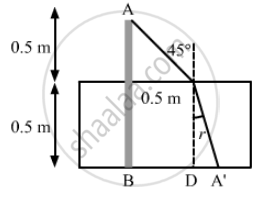Department of Pre-University Education, KarnatakaPUC Karnataka Science Class 12
Advertisement Remove all ads

# A Pole of Length 1.00 M Stands Half Dipped in a Swimming Pool with Water Level 50.0 Cm Higher than the Bed. - Physics

Sum

A pole of length 1.00 m stands half dipped in a swimming pool with water level 50.0 cm higher than the bed. The refractive index of water is 1.33 and sunlight is coming at an angle of 45° with the vertical. Find the length of the shadow of the pole on the bed.

Advertisement Remove all ads

#### Solution

Given,
Length of the pole = 1.00 m
Water level of the swimming pool is 50.0 cm higher than the bed.
Refractive index (μ) of water = 1.33According to the figure, shadow length = BA' = BD + DA'= 0.5 + 0.5 tan r
Using Snell's law:

$Now 1 . 33 = \frac{\sin 45^\circ }{\sin r}$

$\Rightarrow \sin r = \frac{1}{1 . 33\sqrt{2}} = 0 . 53$

$\Rightarrow \cos r = \sqrt{1 -\sin^2 r}$

$= \sqrt{1 - (0 . 53 )^2} = 0 . 85$

$So, \tan r = 0 . 6235$
Therefore, shadow length of the pole  = (0.5)
$\times$ (1 + 0.6235) = 0.81175 m
= 81.2 cm

Concept: Refraction
Is there an error in this question or solution?
Advertisement Remove all ads

#### APPEARS IN

HC Verma Class 11, 12 Concepts of Physics 1
Chapter 18 Geometrical Optics
Q 15 | Page 413
Advertisement Remove all ads

#### Video TutorialsVIEW ALL 

Advertisement Remove all ads
Share
Notifications

View all notifications

Forgot password?